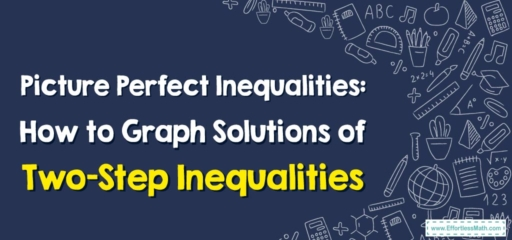# Picture Perfect Inequalities: How to Graph Solutions of Two-Step Inequalities

In today's blog, we're combining two important mathematical skills: solving inequalities and graphing. Specifically, we'll learn how to graph solutions of two-step inequalities.## 1. Two-Step Inequalities and Their Solutions

Two-step inequalities are just like one-step inequalities, but they require two steps to isolate the variable. For instance, to solve the inequality $$2x + 3 > 7$$, you would first subtract $$3$$ from both sides and then divide by $$2$$.

## 2. Graphing Solutions

Graphing solutions to two-step inequalities helps you visualize the range of possible solutions. This can be especially useful when comparing solutions or when working with more complex problems.

## Step-By-Step Guide to Graphing Solutions of Two-Step Inequalities

Now, let’s go over how to graph solutions of two-step inequalities.

### Step 1: Solve the Inequality

Solve the inequality as you would normally. For our example, $$2x + 3 > 7$$, subtract $$3$$ from both sides to get $$2x > 4$$, then divide by $$2$$ to find $$x > 2$$.

### Step 2: Draw a Number Line

Draw a number line that includes the value of your solution and a few numbers above and below it. In our example, you might draw a line from 0 to $$5$$.

### Step 3: Mark the Solution

If your inequality includes “or equal to”, make a filled dot at your solution value. If not, make an open circle. For $$x > 2$$, we’d make an open circle at $$2$$.

### Step 4: Shade the Solution Area

Shade the part of the line that includes your solution. For $$x > 2$$, we’d shade everything to the right of $$2$$.

And that’s it! The graph of the solution to the inequality $$2x + 3 > 7$$ shows that any number greater than $$2$$ would make the inequality true.

By practicing these steps, you’ll develop a strong foundation in both solving and graphing inequalities, skills that are key in many areas of mathematics and science.

Keep practicing, stay curious, and as always, enjoy your journey in the world of math!

This blog post has provided a solid understanding of how to graph solutions of two-step inequalities, complete with a step-by-step guide. Happy graphing!

### What people say about "Picture Perfect Inequalities: How to Graph Solutions of Two-Step Inequalities - Effortless Math: We Help Students Learn to LOVE Mathematics"?

No one replied yet.

X
30% OFF

Limited time only!

Save Over 30%

SAVE $5 It was$16.99 now it is \$11.99